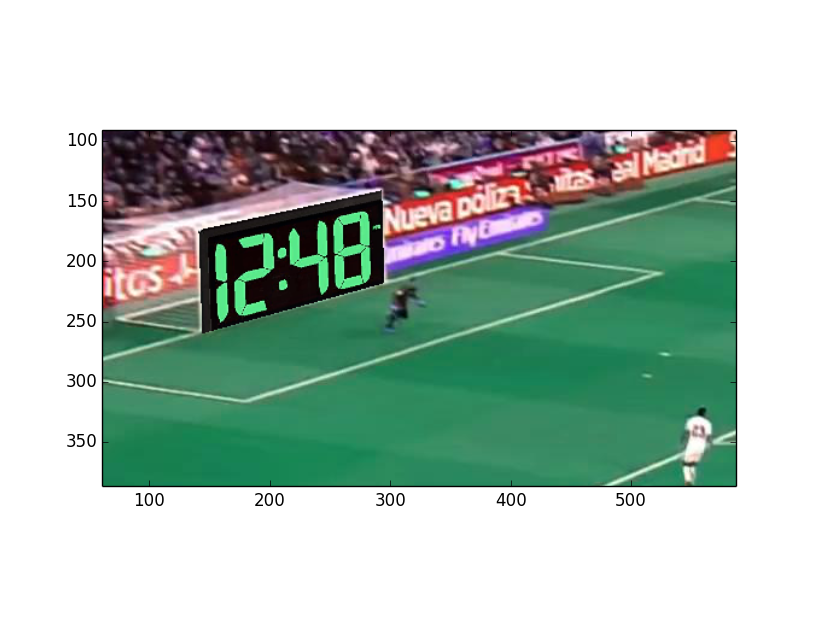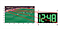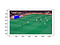# Image Projections for Augmented Reality

Christian Zuniga, PhD

Augmented Reality (or AR) is a system that enhances the real world with a seamless integration of computer-generated perceptual information . Image projections are frequently used in AR to transform an image into the perspective of another image. This projection gives the appearance an object is in a scene when in reality it is not actually there. Figure 1 for example shows a clock projected onto the goal of a soccer field. The clock appears to stand in the area but is not there in reality. This is a modified example from the Perception Course in Coursera from the University of Pennsylvania.Figure 1 The image of a clock projected onto the goal area.

Two separate images made Figure 1: an image of the soccer field (Figure 2), and an image of a digital clock (Figure 3). These will be referred as ‘imgField’ and ‘imgClock’, respectively. A projection matrix H (also called a homography) relates the coordinates of the field image (xF,yF) with coordinates of the clock image (xC,yC).

The projection assumes the image of the clock came from two camera views: one straight on as in Figure 3, and the other from a side as in Figure 1. The pixels in the area of the goal were then replaced by their corresponding pixels in the clock image.

To find the corresponding points, matrix H needs to be found. Image points are first expressed in homogenous coordinates. This is a more useful representation for many computer vision applications . Among other advantages, this representation allows many transformations such as translation, rotation, and scaling to all be expressed in the form of a matrix multiplication. A coordinate (x, y) in an image has homogenous coordinates (lx, ly, l) where l is an arbitrary scalar not equal to zero. A point has multiple homogenous coordinate representations. The points in the soccer field image are represented by (xF, yF, 1) and the points in the clock image by (lxc, lyc, l ). H then relates them by a matrix multiplication.

The direct linear transform method can be used to solve for H. There need to be corresponding points between the two images. These are points of an object that appear in different locations in the images. These corresponding points have to be known beforehand. Since H has only 8 independent variables because of the arbitrary scaling parameter l, a minimum of 4 corresponding points between the images in Figures 2 and 3 are needed. The 4 corner points of the goal shown in Figure 4 will be used. Each point corresponds to a corner point in the clock image, numbered 1 through 4.Figure 4 Corners of goal and of clock image used as corresponding points.

Each of these 4 corresponding points is related by:

All the coordinates are known but not the elements of H. The equations can be arranged to give a least squares problem for the elements of H . Each pair of corresponding points contributes 2 equations after dividing them by l. These are arranged into an 8x9 matrix A to give.

The vector h contains the unknown elements of H. It is found using least squares, which minimizes.

The singular value decomposition (A = USV’) can be used to find h. The columns of V are eigenvectors of A’A and are arranged according their corresponding eigenvalue. Making h equal to the eigenvector having smallest eigenvalue will then minimize the expression. The minimum of J is the smallest eigenvalue of A’A. H is then found by reshaping h to a 3x3 matrix.

Once H is found, it can be used to transform the points in the goal area shown in Figure 5 to their corresponding points in the clock image. Although the transformation can be done both ways, going from field to clock coordinates avoids the possibility of having empty points in the field image. The image contents of the goal area are then replaced with those of the clock, while preserving perspective.Figure 5 Points in goal area will be transformed to corresponding points in clock image.

OpenCV, an open source computer vision library, has built-in functions to find a homography and transform points . However for learning purposes, it is useful to program them directly. Python and Numpy will be used for this example where Numpy is imported as ‘np’. The field image shape is (720,1280,3) and the clock image is (174,334,3).

The function ‘estimateHomography’ calculates the homography matrix using the direct linear method. It takes the corner points of the goal and of clock as input. Then the SVD is used to find the homography.

The function ‘inverse_warping’ then takes the calculated homography and finds the corresponding points of the goal area points in the clock image. The points of the goal area are in a matrix ‘sample_pts’ and found separately. For example, the openCV function ‘pointPolygonTest()’ could be used to find them. Finally the contents of the field image, in the goal area, are replaced with the contents of the corresponding points in the clock image and the modified image returned.

References

 E.R. Davies, “Computer Vision”, 5th edition, Academic Press 2018

 Solem “Programming Computer Vision with Python”, O’Reilly 2012

Christian Zuniga has worked as a lecturer in San Jose State University in data mining. He worked in OPC modeling and is interested in machine learning.

## More from Christian Zuniga

Christian Zuniga has worked as a lecturer in San Jose State University in data mining. He worked in OPC modeling and is interested in machine learning.